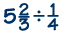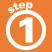Home    |    Teacher    |    Parents    |    Glossary    |    About UsHere are the steps for dividing mixed numbers.

1. Change each mixed number to an improper fraction.
2. Multiply by the reciprocal of the divisor, simplifying if possible.
3. Put answer in lowest terms.
4. Check to be sure the answer makes sense.

Click on each step below to see an example.Homework Help | Pre-Algebra | FractionsEmail this page to a friendSearch·  Definitions·  Reducing fractions·  Adding and subtract-     ing fractions·  Multiplying fractions·  Dividing fractions·  Adding and subtract-     ing mixed numbers·  Multiplying     mixed numbers·  Dividing     mixed numbersFirst Glance In Depth Examples WorkoutDividing mixed numbers